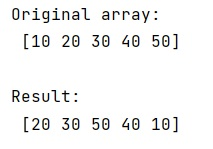# How to rearrange array based on index array?

Learn, how to rearrange array based on index array in Python?
Submitted by Pranit Sharma, on February 08, 2023

NumPy is an abbreviated form of Numerical Python. It is used for different types of scientific operations in python. Numpy is a vast library in python which is used for almost every kind of scientific or mathematical operation. It is itself an array which is a collection of various methods and functions for processing the arrays.

## Rearranging array based on index array

Suppose that we are given a numpy array and an array of indices. We need to arrange the elements of this numpy array according to the index array.

For example, if the index array has 0 at the 3rd position then the 0th element of the numpy array will be shifted to the 3rd position.
There is a very easy approach to this problem, We will simply use the indexing technique. We will index the original array with the array of indices.

Let us understand with the help of an example,

## Python code to rearrange array based on index array

```# Import numpy
import numpy as np

# Creating a numpy array
arr =  np.array([10, 20, 30, 40, 50])

# Display original array
print("Original array:\n",arr,"\n")

# Creating an array of indices
ind = [1, 2, 4, 3, 0]

# Re-arranging array
res = arr[ind]

# Display Result
print("Result:\n",res,"\n")
```

Output: